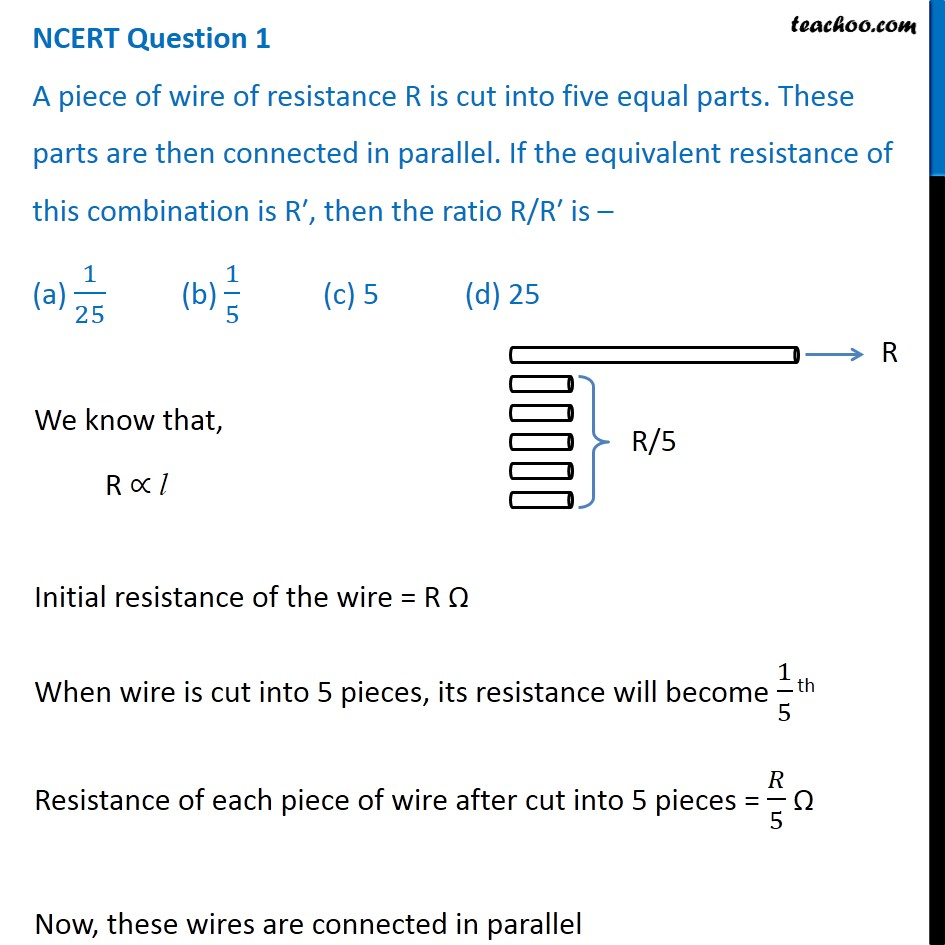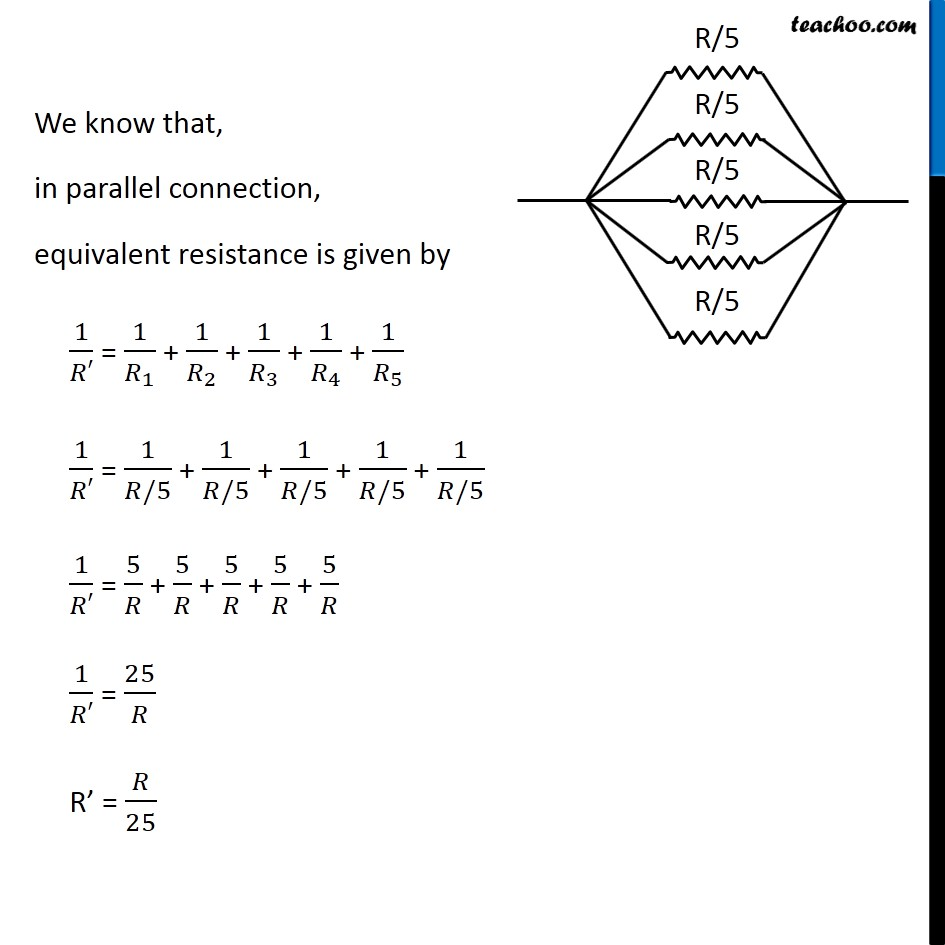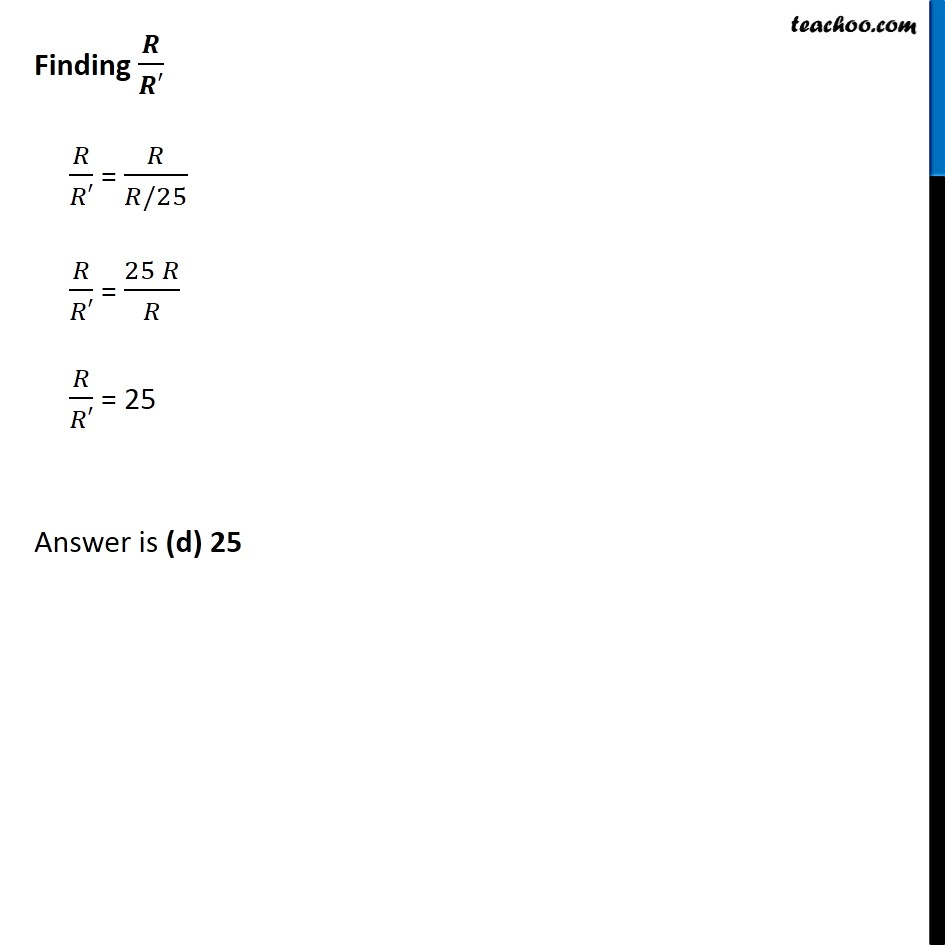NCERT Questions

Class 10
Chapter 12 Class 10 - Electricity

## A piece of wire of resistance R is cut into five equal parts. These parts are then connected in parallel. If the equivalent resistance of this combination is R′, then the ratio R/R′ is – (a) 1/25 (b) 1/5 (c) 5 (d) 25Learn in your speed, with individual attention - Teachoo Maths 1-on-1 Class

### Transcript

NCERT Question 1 A piece of wire of resistance R is cut into five equal parts. These parts are then connected in parallel. If the equivalent resistance of this combination is R′, then the ratio R/R′ is – (a) 1/25 (b) 1/5 (c) 5 (d) 25 We know that, R ∝ l Initial resistance of the wire = R Ω When wire is cut into 5 pieces, its resistance will become 1/5 th Resistance of each piece of wire after cut into 5 pieces = 𝑅/5 Ω Now, these wires are connected in parallel We know that, in parallel connection, equivalent resistance is given by 1/𝑅′ = 1/𝑅_1 + 1/𝑅_2 + 1/𝑅_3 + 1/𝑅_4 + 1/𝑅_5 1/𝑅′ = 1/(𝑅/5) + 1/(𝑅/5) + 1/(𝑅/5) + 1/(𝑅/5) + 1/(𝑅/5) 1/𝑅′ = 5/𝑅 + 5/𝑅 + 5/𝑅 + 5/𝑅 + 5/𝑅 1/𝑅′ = 25/𝑅 R’ = 𝑅/25 Finding 𝑹/𝑹′ 𝑅/𝑅′ = 𝑅/(𝑅/25) 𝑅/𝑅′ = (25 𝑅)/𝑅 𝑅/𝑅′ = 25 Answer is (d) 25